# 3D Rotations in Processing (Vectors, Matrices, Quaternions)

This tutorial introduces how to rotate objects in 3D beyond Euler angles; to do this, it looks at the basics of matrices and quaternions. What follows is math heavy, so a robust artistic imagination will be valuable once we dig in.

The approach we use is that of an excavation. We’ll recreate functionality already implemented behind the scenes in Processing and risk statements of the obvious so as to make the implicit explicit. We undertake these redundant tasks to better understand how rotation works not just in Processing but across 3D graphics engines and to build new capabilities on top of hopefully improved foundations.

A few notes beforehand:

• This tutorial assumes enough familiarity with Processing to know where to find documentation for new concepts. For example, a prior acquaintance with vectors is encouraged, and chapter 1 of Dan Shiffman’s Nature of Code provides a good introduction.
• Processing version 3.3.6 was used for the example code. A warning for PC users: In the past, working in 3D with Processing caused significant lag.
• For those who are looking for a library that has already implemented these concepts, a few are glMatrix (JavaScript), Physically Based Rendering (C++), Three (JavaScript), and Toxiclibs (Processing). They have served as references for the code that follows.

# Review of 2D Vectors

For any task in 3D, we can revisit what we know in 2D, then work outward from there. Introductions to vectors often assert that 1.) they are not positions in space and 2.) they have magnitude (or length) and a heading (or direction). These two assertions are easy to forget or dismiss, since, after the preliminaries, we use vectors to record positions with 2 or 3 `float`s or `double`s (none of which are heading or magnitude). As the following sketch demos,

we could build our vectors with fromAngle were we so inclined. It creates a unit vector with a magnitude of 1 by assigning the cosine of theta to x and the sine of theta to y (i.e., by converting from polar to Cartesian coordinates). PVector.random2D picks a random number between 0 and `TWO_PI` then supplies it to `fromAngle`.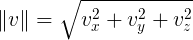The magnitude of v equals the square root of the sum of its components squared.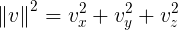The intermediate step, magSq, merits recognition due to the cost of sqrt.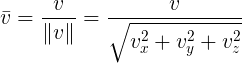A unit vector is created through normalization, by dividing its components by its magnitude.

To read the heading of a pre-existing 2D vector, heading finds the arctangent `atan2` of the vector’s y and x. To find the magnitude of a vector, we use the Pythagorean theorem. To set the magnitude of a unit vector, we multiply each component by the magnitude. For vectors not of unit length, we first normalize by dividing by the magnitude, then multiply by new magnitude, as the function setMag does.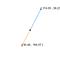Setting a vector’s magnitude by a negative value will reverse its direction.

When negative values are passed to `setMag` or limit, the `PVector`’s direction will be reversed.

In Euclidean geometry, a magnitude is generally assumed to be positive, with zero being the lower bound.

To summarize, fields listed at the top of a Processing class (`x`, `y` and `z`) do not always correspond with our conceptualization of a mathematical entity. These fields are often the minimum inputs needed for the metamorphoses which motivate our learning the math in the first place.

Why not define a `class Vec { float heading, magnitude; }` then? Although a `float` suffices to describe a 2D rotation, 3D requires more complex objects. Those aforementioned metamorphoses come at a cost: square root and trigonometric functions (cosine, sine, arctangent) are expensive to calculate. And while computer processing power increases, making these costs trivial, so too does the thirst for spectacle (more particles, light bounces, greater fidelity). The x, y, and z components of a vector are akin to latent potential, actualized when an action is called for… that so happen to match those used by a spatial coordinate.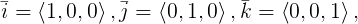The orthonormal basis is i < 1, 0, 0 >, j < 0, 1, 0 >, k < 0, 0, 1 >.

This returns us to the first assertion: a vector alone is not a point, but can serve as one given a frame of reference: an orthonormal basis. In the demo above, before the animation has begun, the vector `position` has traveled from the origin in the top left corner with reference to the horizontal axis i, where a positive value moves screen right and a negative value to screen left; the vertical axis, j, where a positive value moves screen down and a negative value screen up; and k, where a negative value recedes toward the horizon.

Given any two axes of this basis, we can derive the third with the cross product (or outer product), cross in `PVector` .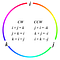Given any two axes of the basis, the third can be found from the cross product. Crossing two unit vectors does not necessarily yield a unit vector.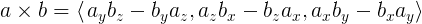a cross b = < a.y * b.z — b.y * a.z, a.z * b.x — b.z * a.x, a.x * b.y — b.x * a.y >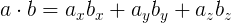The dot product of vectors a and b is the sum of the product of their components.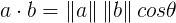The dot product of two vectors is cosine the angle between them multiplied by their magnitudes.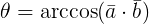The angle between unit vectors a and b is arccosine of the dot product of the normalized vectors.

The relationship between a basis and rotation becomes clearer with the dot (or inner) product. This is the sum of the product of each vector’s corresponding components. If the vectors are normalized, the result equals the cosine of the angleBetween them. Rotation functions often will start with `dot` because of this.

`acos` is more sensitive to the angle’s range than we might first imagine. If we use 0 .. `TWO_PI`, `acos` may return not the angle, but its supplement, 360 - the angle; . If we use `-PI` .. `PI`, `acos` returns the positive or negative angle.

There’s another hair in the soup. Suppose we `rotate` a vector around an origin, a rotation around the z axis. If this vector represents a person walking in circles around a pole, the meaning of ‘forward’, ‘right’ and ‘up’ will be different for that person in local space than in world space as perceived through a virtual camera.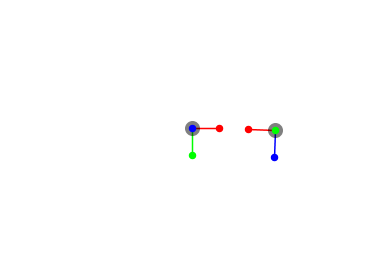The local space of a rotating point compared to global space at the origin.

This is clearer when we switch to 3D and pan the camera around the origin.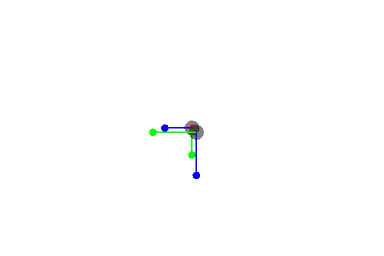The local space of a rotating point compared to global space at the origin.

The same point in space can be represented by any number of vectors depending on the base, which is why Processing has screen and model functions, and why more complex engines distinguish between local and global position, rotation and scale.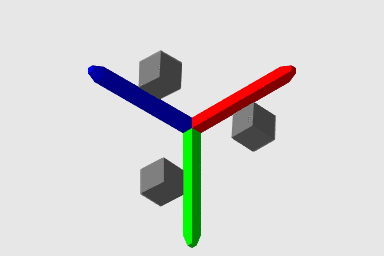Processing’s chirality, viewed in an orthographic camera from <1, 1, 1>.

Orthonormal vectors (orthogonal and normalized, or perpendiculars of unit length) are no less arbitrary for world space than they are for local space. In a photo editing program like Gimp, positive y leads down, while in a 2D platformer, it leads up. In the 3D modeling software Blender, the z axis is the vertical axis while the y axis indicates depth; the two axes are flipped in Unity.

Coordinate systems fall under the left- or right-handed rule, which describe whether rotations travel clockwise (CW) — left-handed — or counterclockwise (CCW) — right-handed. To maintain consistency between 2D and 3D, Processing flips the y axis of the underlying 3D renderer, OpenGL.

Before moving on, we note that, when calculating local bases, the cross product of two unit vectors is not a unit vector, as seen below:

A sample result would be `c [ -0.28 , -0.02 , -0.12 ] |c| 0.301 n [ -0.92 , -0.06 , -0.39 ] |n| 1.000 `. The area formed by cross products between two vectors is a parallelogram in 2D, and a parallelepiped in 3D. Nor does linear interpolation (lerp)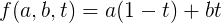Linear interpolation from a to b by a step is a multiplied by the complementary step plus b multiplied by the step.

between two normalized vectors result in a normalized vector. This is crucial when rotating from one angle to another instead of rotating by an increment.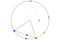c (purple) is interpolated linearly from a (orange) to b (teal). the magnitude of a and b are equal. d (magenta) is interpolated from the angle of a to the angle of b.

A workaround in 2D is to interpolate between the headings of the two vectors, preferring the shortest distance (torque minimization); the problematic case here is `delta == 0`, when the two points are 180 degrees apart and neither CW nor CCW is shorter. We can see the distance between the purple and magenta points as they travel from the start point, in orange, and the end point, in blue, one along a chord the other along an arc. They both reach the destination in the same amount of time, but the magenta point travels a greater distance. If linear interpolation had to cover the same distance as angular interpolation in the same time, how would that influence the speed of the purple point? Meanwhile, the magenta keeps constant angular velocity.

We raise these issues now because a 3D rotation mandates that the collection of points which compose a model be rotated in concert while maintaining proportion; a mathematical entity called the quaternion resolves this issue.

# Creating A Vector from Azimuth and Inclination

Leaping into 3D from the 2D refresher, we first search for the equivalent to `fromAngle`. There is none. What we learn from Dan Shiffman’s video on Spherical Geometry, is that a vector can be created in with spherical coordinate system using azimuth, corresponding to longitude (meridian) on a globe, and inclination, corresponding to latitude.

We try to compensate for the flipped y axis by using `-phi` rather than `phi`. Since `PVector` represents both 2D and 3D vectors, we aim for consistency between the original `PVector.fromAngle` and the new by fudging the math formula above. The same result is returned when `phi` is `-HALF_PI` (-90 degrees).

The range of the azimuth is twice that of the inclination; we initialize twice as many longitudes as latitudes. When specifying the inverse maximum number of longitudes and latitudes (`invLongitudes = 1.0 / float(longitudes); invLatitudes = 1.0 / float(latitudes — 1);`) so that in draw we can describe our progress along the sphere as a percentage with `ipercent` and `jpercent`, one is subtracted from latitudes. Longitude is periodic; to travel all the way around the globe is to reach one’s point of departure. Latitude is not; there is no more North than the North Pole. Starting the count at 0, 100% progress is at iteration n - 1. This subtraction leads to overlapping points at a pole, hence the z-fighting, but is helpful should we wish to connect these points into edges and later, faces. Lastly, we color each point with hue, which like longitude is periodic. Were we to ease between colors by a different method, there would be a seam.

The upshot of the last paragraph is that we are treating vectors as points, not directions. To demo how our new `fromAngle` function can assist us with directions, in the following sketch we construct the arguments for directionalLight (or spotLight).

# Rotations of a Vector on X, Y and Z Axes

Suppose we’ve already got a vector, maybe constructed with Cartesian coordinates or retrieved from a `PShape`. There are no axis-specific rotations for 3D vectors. There are general rotateX and rotateY functions, which we typically place between pushMatrix and popMatrix (more on those later)… there are also PShape and PMatrix3D versions… there’s a `rotate` for 2D vectors… a rotation can be applied to a vector with `PMatrix3D`’s mult. If those suffice, this section can be skipped.

Because the `PVector` class is not marked as `final`, it can be `extend`ed. We can write new methods, including `overload`s (functions with the same name, but a different list of parameters), to the child- or sub-class of `PVector`. We can also `override` functionality. For example, `toString` is commonly overridden, but generally it is not advisable to do so without careful study of the parent function the override replaces. As a child of `PVector`, a `Vec3` can be passed into any function that would accept a `PVector`; the child should behave like its parent anywhere in the Processing engine.

Methods which change the vector’s components honor chainability with `return this;`, meaning we can chain together .method calls, e.g., `v.normalize().rotate(PI).translate(0.0, 50.0);`. To test drive this new code, we use the following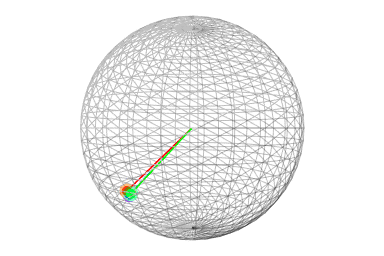Rotation of a point <50, 50, 50> around i (red), j (green) and k (blue).

Introducing matrix notation now will prepare us for tougher cases. In a rotation matrix, each column represents i, j and k — the basis of the vector — with the unused fourth column being translation. Each row represents the axis x, y, and z components, with an unused w component.

For each rotation, the vector component corresponding to the axis around which we rotate remains unchanged. In `rotateX`, for example, the i column and the x row are all 0s except for the 1 where they cross.

These rotation matrices are applied to a vector through the dot product. Between two vectors, we safely assumed that each had the same number of components; how do we multiply x, y and z against 4 columns and rows? The vector is converted to a homogeneous coordinate by tacking on a w, 1, then represented as a column. After finding the dot product, we convert from a homogeneous coordinate to a vector by dividing its components by w (w divided by itself is one).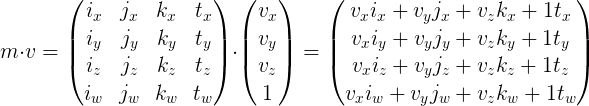A transformation is the dot product of a matrix m and homogeneous coordinate v, the sum of the products of v’s components with the corresponding component in m’s columns.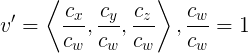The transformed point v equals the x, y and z components of homogeneous coordinate c divided by w. the w, now equal to the identity 1, is discarded.

For those curious about homogeneous coordinates, Squirrel Eiserloh has given a talk at GDC, “Math for Game Programmers: Understanding Homogeneous Coordinates.”

Why bother recreating these rotation functions for vectors? Consider a 3D model, perhaps described in a .obj file, imported into Processing via `loadShape` and stored in a `PShape`. It is more complicated to track the neighboring faces of a face, the half-edges contained by a face, and the vertices contained by an edge than it is to create the illusion of unity by calculating one transformation matrix then applying it to all the vertices. If we need to rotate only a handful of vectors, these functions allow us to do so without unnecessary multiplications and additions, and without pushing and popping matrices onto and off of the stack.

# Rotations of a Vector by Axis and Angle

Rotations around i, j and k will not suffice to orient models in space, primarily due to gimbal lock. Changing the order in which we perform rotations does not alleviate the issue. If we want to rotate a vector around an arbitrary axis by an angle, we return to matrix notation,

where u is the rotation axis. A few patterns stand out. Reading the columns from left to right, we cycle through these operators between terms: + + - ,- + + , + - + . In each entry, the axis component corresponding to its row is multiplied by that of its column; for example, in column 1 (j, up in local space), row 2 (z row), axis y is multiplied by axis z. This correlates with the diagonal, where the axis component is squared. Also on the diagonal, cosine theta is added, rather than +/- sine theta multiplied by the component excluded from the row-column pattern.

This looks opaque, and its bulkiness may dissuade us from trying to understand matrices further. For comparison, let’s follow the steps to derive Rodrigues’s rotation formula using vector operations instead:

1. project a point onto an axis;
2. find the elevation of the point, the component perpendicular to the axis;
3. find the plane of rotation about the axis;
4. extend a point from the plane of rotation onto the plane containing the original point.

## Project The Point Onto The Axis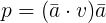The projection of point v equals the dot product of v and axis a multiplied by a.

In the image to the left, the point is orange and the axis is blue. The pink dot is the projection of the point onto the axis; the shadow cast by the point, figuratively speaking. The axis is a direction; not only does its magnitude not matter, we have less work to do if the axis remains normalized. Whether or not the pink dot lays on the line segment we use to display the axis matters more because of what it signifies: the size of the angle between the axis and the orange point.

## Find the Elevation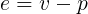The elevation of point v equals v minus its projection onto axis a.

We switch to an orthographic projection so as to see the relation between these vectors without distortion. The perpendicular, or elevation, is lime green.

## Find the Plane of Rotation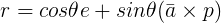The plane of rotation r equals cosine theta multiplied by the elevation plus sine theta multiplied by the axis a crossed with projection p.

The point where the elevation (lime green) joins the origin at a right angle will lie on the circumference of the plane of rotation (purple). The distance of this point from the circle’s center will correspond to the magnitude of the projection (pink).

## Extend a Rotated Point to the Original’s Plane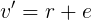The rotation of v around axis a equals the rotation r plus the elevation.

The last step is to add the projection (pink) to the circumferential point (purple). As can be seen, a conical or cylindrical form is suggested by the above.

With two approaches to axis-angle rotation under our belt, we can compare them. The vector approach is easier to understand, but is drawn out across many steps, and we can’t always be sure that a prior step has been completed before moving on to the next which depends upon it (it is wasteful to keep checking if the axis is normalized, for example).

As an example of how these functions can be incorporated into a more involved sketch, left, we store a a sphere of vectors in an array; they rotate around an axis by an angle dictated by their array index. A modulo operator can be used to set every other latitude (or longitude) to a different radius from the sphere’s center than the rest. The axis is itself rotated.

# 4 x 4 Matrices

As mentioned, Processing already has a 4 x 4 matrix, `PMatrix3D` with 16 floats named as in the figure to the left. When we use the sketch renderer’s `pushMatrix` and `popMatrix`, we are pushing and popping matrices onto and off of a stack data structure. A stack is a deceptively literal name— just like a stack of dirty dishes, the first item pushed onto the stack is the last to be popped off and addressed (LIFO, as opposed to a queue, which is FIFO, first-in-first-out). Just as we can’t pull any dish from the middle without upsetting the stack, neither can we access an element in the middle of a digital stack (unlike, say, an indexed array). This explains why every `pushMatrix` must be matched by a `popMatrix`; too many pushes in a `draw` call and the stack overflows, too many pops and the stack tries to remove nothing from an empty stack.

The `PMatrix3D` class presents a few obstacles, though: it lacks documentation; it is marked `final` and thus cannot be customized. Matrices in `PShape`s cannot be set directly. Rotation matrices are not separated from translation and scale matrices, meaning a shape’s location cannot be changed without changing the pivot point of the underlying geometry.

## Looking Toward A Point

Two key interactions between vectors and matrices are multiplication (already in the `PMatrix3D` class) and the orientation of one shape to look at another. We’ll place these in the `Vec3` class.

Ambiguity arises regarding how these functions are named and used. Because matrix multiplication is not commutative, and v * m is not defined, this function is sometimes called `applyMatrix` .

Some `lookAt` functions assume that the look direction (forward or k in local space) `PVector.sub(origin, target).normalize();` has already been calculated outside the function and passed in as the second `PVector`. In the code above, the generated matrix is applied not to the pivot itself, but rather intended for application to the vertices of the geometry surrounding that pivot. To see `lookAt` in action, we use the following

The next step is to learn how to ease between rotations. For that, we’ll introduce quaternions.

# Quaternions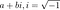A complex number is a real part, a, added to an imaginary part, b.Hamilton’s discovery.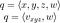A quaternion can be described with four components, x, y, z and w; or as an imaginary vector v and a real part w.

Quaternions were invented in 1843 by William Hamilton, who was so inspired by his discovery that he carved the formula into a bridge nearby. Just as a complex number in 2D contains a real and imaginary component, a quaternion in 4D contains three imaginary components, x, y and z (sometimes a, b and c), and a real component, w (sometimes d). As with vectors before, there’s a gap between how we understand quaternions and what we see in code. A quaternion contains a vector, but is not simply a vector with an extra letter.

## Converting from Axis-Angles, Applying To Points

While the above gloss may keep us from misunderstanding quaternions, it doesn’t help us with how to use them. The formula to create a quaternion from an axis-angle,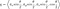A quaternion’s imaginary parts are the normalized axis a multiplied by sine half theta. Its real part is cosine half theta.

is a first step, keeping in mind that, following an operation which changes the magnitude of a quaternion, it should be normalized.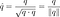A unit quaternion is normalized by dividing the quaternion by its magnitude, or the square-root of its dot product with itself.

The challenge after this is to decide which interactions with the other data types encountered above — points (vectors), directions (vectors), Euler angles (scalars), matrices and other quaternions — are a priority.

We’ll start by coding some essentials, scalar operations, the axis-angle conversion and multiplication with a vector. This will give us enough to visualize the code.

Regarding equality, quaternions represent 720 degrees of rotation, not 360. If we create quaternions from axis-angles, we might be surprised when those we assumed to be equal are not.

## Easing

We can now synthesize what we learned from rotating by an amount in 3D with what we learned rotating from one angle to another in 2D.

Common to any implementation of `slerp`, is how to deal with rotations where two points are 180 degrees apart, where neither CW nor CCW is shorter. Our primary source is the Three.js approach. There’s plenty of variation, though, based on which inverse trigonometry functions (`acos`, `asin`, `atan2`) are used, when to check if the angle supplied to them is in-bounds and when to revert to the cheaper linear interpolation.

Game developers Jonathan Blow (of Braid and The Witness) and Casey Muratori (of Handmade Hero) have made some key observations on quaternions and `slerp`, which Blow wrote about in the article “Hacking Quaternions". Since quaternions rotate around a four-dimensional unit sphere, we can `lerp` between two quaternions then `normalize` to promote the path of travel from a chord to an arc. If we add a `lerp` and naive `nlerp` to our code, we can compare these three possibilities.

Linear interpolation (`lerp`) is shown in green; normalized linear interpolation (`nlerp`); in orange; `slerp`, in purple. `lerp` demonstrates the distortion of a rotating point when quaternions are not normalized. Like `slerp`, this naive `nlerp` travels on an arc, but doesn’t minimize torque or preserve velocity.

Blow next developed a correction function, which augmented the `step` supplied to `nlerp` so as to approximate the speed of `slerp`, then added a fast inverse square-root function to speed up the required normalizations. We won’t implement that portion here; interested readers may reference Blow’s article and Muratori’s video on double cover.

## Converting To and From Matrices

Given the centrality of matrices to Processing, we’ll focus on that conversion next. First, we look at the abstract notation. As with axis-angle rotations, searching for patterns can help us understand this enough to debug the code. On the diagonal, two quaternion components which do not correspond with the row-column are squared, summed, multiplied by 2 then subtracted from 1. The transposition of each cell outside the diagonal is the same except for a flipped sign. For example, in (1, 0) qx qy is added to qz qw, then doubled; in (0, 1) they are subtracted.

We’ll need to test both the quaternion-vector multiplication and matrix conversion to make sure that immediate shapes and retained shapes work.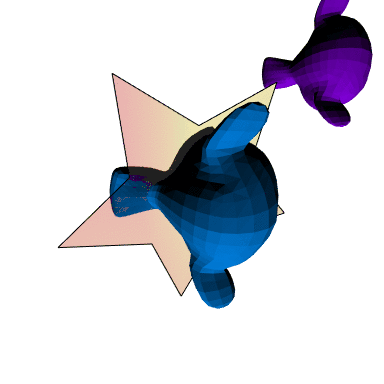A quaternion rotation applied to an immediate shape (star), retained shape (blue Suzanne), tested against a control shape (purple Suzanne).

Because a `PShape` does not allow its matrix to be set, we `reset` to the identity matrix then multiply with `applyMatrix`. To convert from a matrix to a quaternion, we use the following, submitted by Christian to Euclidean Spaces.

At the core of conversion is the matrix trace, the sum of its diagonal.
Other implementations try to limit the use of square root, but the above has the advantage of clarity and brevity. Underneath Processing math functions are those of Java’s `Math` library. One function Processing doesn’t wrap is `signnum`. By multiplying each imaginary component by the result of `signnum`, we ensure that the quaternion has the same sign as the rotation matrix. We can test the conversion in the console.

With a matrix conversion in place, a quaternion `lookAt` function is now easy to make: given an origin, target, and an up direction, find i, j and k. Then supply them to `set`. We’ll conclude with these conversions. Should we need to research more than what’s covered in this tutorial, Euclidean Spaces is a handy reference.

# Conclusion

Now that some basics of rotation are taken care of, what’s available to us? This enables us to create `Transforms` which track rotation data for 3D objects in our sketch without need of `pushMatrix` and `popMatrix`. If we add Euler angle conversions to our code, we could import and interpret a BioVision Hierarchy (.bvh) file then pass it on to either matrices or quaternions. And even more opportunities open up should we choose to work more directly with OpenGL.